# 程序员那些必须掌握的排序算法(上)

#### 1.冒泡排序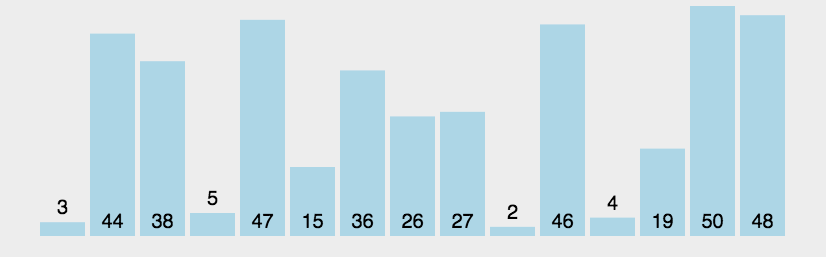```@Test
public void bubbleSort() {
int[] arr = { 3, 44, 38, 5, 47, 15, 36, 26, 27, 2, 46, 4, 19, 50, 48 };
// 统计比较次数
int count = 0;
// 第一轮比较
for (int i = 0; i < arr.length - 1; i++) {
// 第二轮比较
for (int j = 0; j < arr.length - 1 - i; j++) {
if (arr[j] > arr[j + 1]) {
// 交换位置
int temp = arr[j];
arr[j] = arr[j + 1];
arr[j + 1] = temp;
}
count++;
}
}
System.out.println(Arrays.toString(arr));
System.out.println("一共比较了:" + count + "次");
}
```

```[2, 3, 4, 5, 15, 19, 26, 27, 36, 38, 44, 46, 47, 48, 50]

```

```@Test
public void bubbleSort() {
int[] arr = { 3, 44, 38, 5, 47, 15, 36, 26, 27, 2, 46, 4, 19, 50, 48 };
// 统计比较次数
int count = 0;
for (int i = 0; i < arr.length - 1; i++) {
boolean flag = true;
for (int j = 0; j < arr.length - 1 - i; j++) {
if (arr[j] > arr[j + 1]) {
// 交换位置
int temp = arr[j];
arr[j] = arr[j + 1];
arr[j + 1] = temp;
flag = false;
}
count++;
}
if(flag) {
break;
}
}
System.out.println(Arrays.toString(arr));
System.out.println("一共比较了:" + count + "次");
}
```

```[2, 3, 4, 5, 15, 19, 26, 27, 36, 38, 44, 46, 47, 48, 50]

```

#### 2.选择排序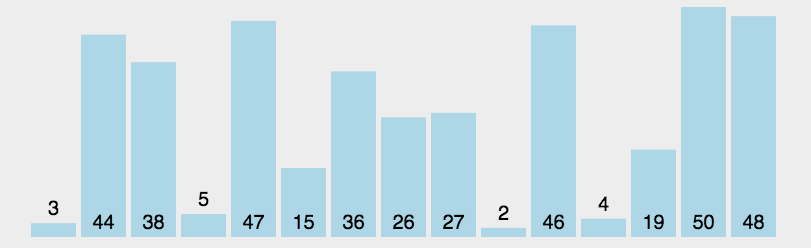```@Test
public void SelectionSort() {
int[] arr = { 3, 44, 38, 5, 47, 15, 36, 26, 27, 2, 46, 4, 19, 50, 48 };
for (int i = 0; i < arr.length - 1; i++) {
int index = i;
for (int j = 1 + i; j < arr.length; j++) {
if (arr[j] < arr[index]) {
index = j;// 保存最小元素的下标
}
}
// 此时已经找到最小元素的下标
// 将最小元素与前面的元素交换
int temp = arr[index];
arr[index] = arr[i];
arr[i] = temp;
}
System.out.println(Arrays.toString(arr));
}
```

```[2, 3, 4, 5, 15, 19, 26, 27, 36, 38, 44, 46, 47, 48, 50]
```

#### 3.插入排序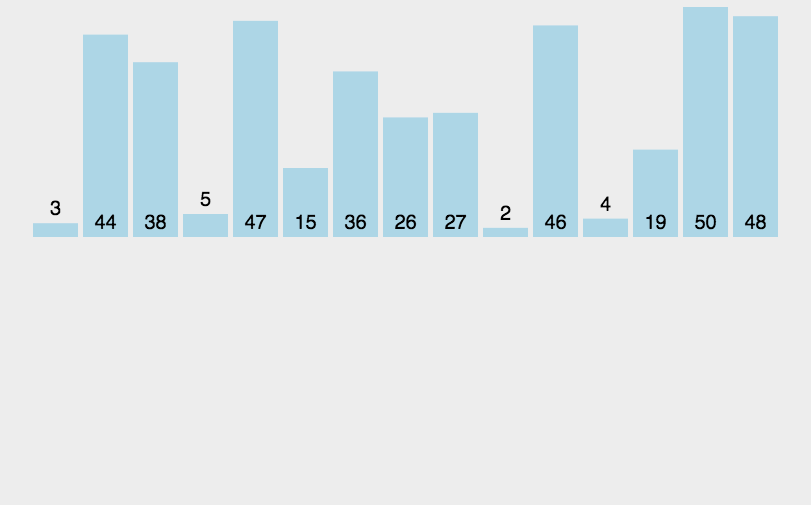```@Test
public void InsertionSort() {
int[] arr = { 3, 44, 38, 5, 47, 15, 36, 26, 27, 2, 46, 4, 19, 50, 48 };
for (int i = 1; i < arr.length; i++) {
// 定义待插入的数
int insertValue = arr[i];
// 找到待插入数的前一个数的下标
int insertIndex = i - 1;
while (insertIndex >= 0 && insertValue < arr[insertIndex]) {
arr[insertIndex + 1] = arr[insertIndex];
insertIndex--;
}
arr[insertIndex + 1] = insertValue;
}
System.out.println(Arrays.toString(arr));
}
```

```[2, 3, 4, 5, 15, 19, 26, 27, 36, 38, 44, 46, 47, 48, 50]
```

#### 4.希尔排序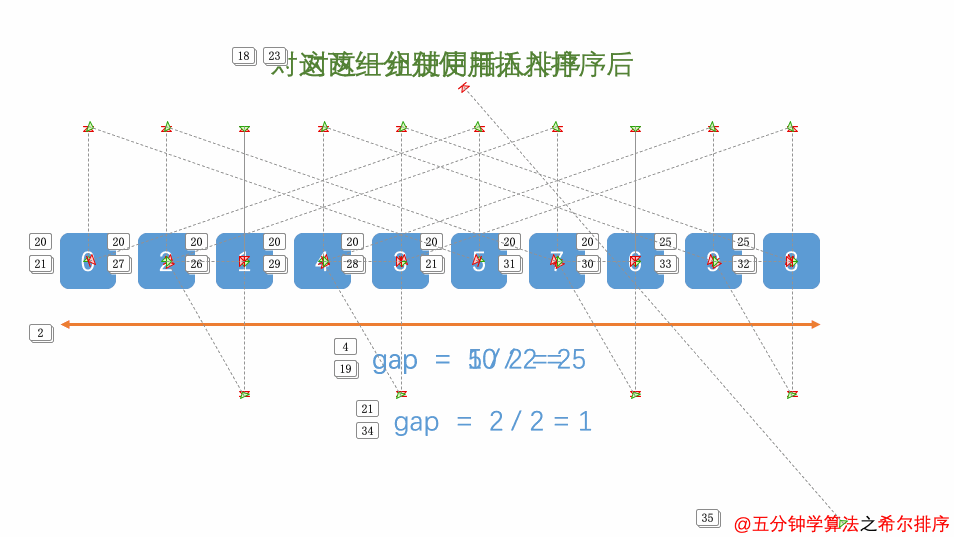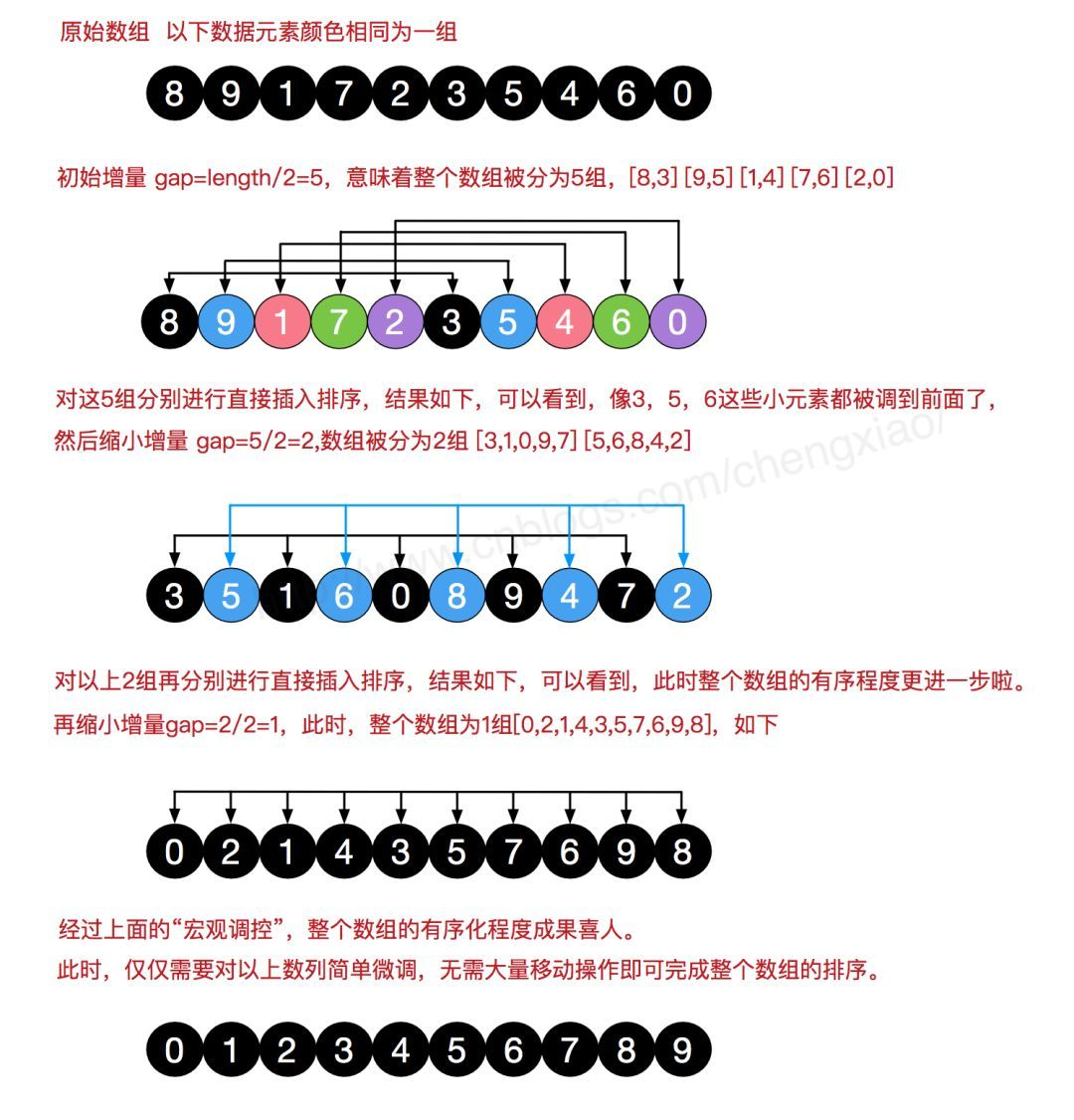```@Test
public void ShellSort() {
int[] arr = { 3, 44, 38, 5, 47, 15, 36, 26, 27, 2, 46, 4, 19, 50, 48 };
for (int gap = arr.length / 2; gap > 0; gap /= 2) {
// 对数组元素进行分组
for (int i = gap; i < arr.length; i++) {
// 遍历各组中的元素
for (int j = i - gap; j >= 0; j -= gap) {
// 交换元素
if (arr[j] > arr[j + gap]) {
int temp = arr[j];
arr[j] = arr[j + gap];
arr[j + gap] = temp;
}
}
}
}
System.out.println(Arrays.toString(arr));
}
```

```[2, 3, 4, 5, 15, 19, 26, 27, 36, 38, 44, 46, 47, 48, 50]
```